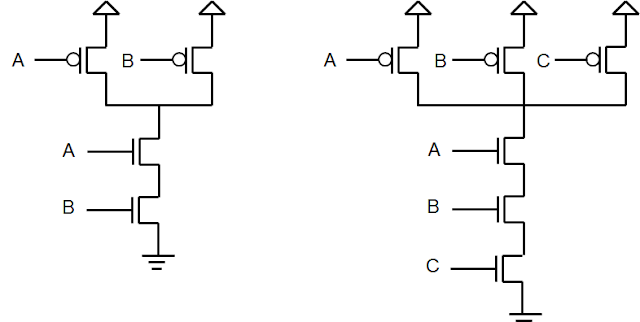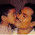## April 08, 2018

### Leakage Power: Input Vector Dependence

Leakage Power of a standard cell depends on various transistors parameters like the channel length, threshold voltage, substrate or the body bias voltage etc. Apart from these physical parameters, leakage power also depends upon the input vector applied.

Consider a 2-input NAND gate and a 3-input NAND gate. Can you arrange the input combinations: (AB = 00, 01, 10, 11 for a 2-input NAND gate), and (ABC = 000, 001, 010, 011, 100, 101, 110, 111 for a 3-input NAND gate) in increasing order of leakage current, with a word of two about the logical reasoning behind it?

Note that the order of transistors in a stack matters here.2-input NAND and 3-input NAND Gates

#### 4 comments:

1.Since the leakage current is more when transistor is ON (larger area) compared to OFF transistor, the minimum leakage input pattern should maximize the number of disabled (off) transistors in all stacks across the circuit.
Here in NAND gates, stacking is with NMOS transistors.

For a 3 input NAND gate, the input vector pattern goes like goes like 000, 011, 001, 010, 100, 101, 110, 111 in the increasing order of leakage current, briefly explained below.

0 0 0 Three OFF Transistors (NMOS) in stack => Least Leakage current
0 1 1 One OFF transistor, But since A (NMOS) is OFF, leakage current passing through B and C should be negligible. Should be close to least Leakage Current
0 0 1 Two OFF transistors in stack
0 1 0 Two OFF transistors, but since A (NMOS) got OFF, leakage current passing through B should be less
1 0 0 Two OFF transistors in stack
1 0 1 One OFF transistor, But since B (NMOS) is OFF, leakage current passing through C is negligible.
1 1 0 One OFF transistor
1 1 1 Zero OFF transistor => Most leakage current

Similarly for 2 input NAND gate, its 00, 01, 10, 11.

1.Also, Shouldn't 001 and 010 take less leakage power than 011? Because only 1 nmos transistor is ON whereas 011 has 2 transistors ON.

2.Can you please explaint why the leakage is more when the transistor is on. YOu mentioned, "higher area". I dont get it.

3.Leakage current happens, when Ioff is not zero.
Which means Temp = 0 , or Vds = 0
If you calculate for a 2 input nand

A=0 B=0, then Vds(A) = VDD & Vds(B) = 0
A=0 B=1, then Vds(A) = VDD & Vds (B) does not matter as it is not off
A=1 B=0, then Vds(A) = does not matter & Vds(B) = VDD-Vth
A=1 B=1 , no leakage as it is all ON

So for 2-input NAND the highest leakage is for 00 / 01, and the least leaky is 11